## Pages

`“Life is like riding a bicycle.  To keep your balance you must keep moving.”–Albert Einstein`

## Sunday, October 26, 2008

### AP Physics B & C- Answers to Free Response Practice Questions on Newton’s Laws

In the post dated 24th October 2008 two free response practice questions on Newton’s laws were given. As promised, I give below model answers along with the questions:(1) Fig. 1 shows a small block of mass M1 placed on the rough inclined surface of a large wedge shaped block of mass M2 which is at rest on a rough horizontal table top. The coefficients of static friction and kinetic friction (between M1 and M2) are respectively μs and μk. Now answer the following questions (a), (b) and (c):

(a) Block M1 is just going to slide down. Draw the free body diagram for block M1 showing and identifying all the forces acting on it. (Represent M1 by a dot).

(b) What is the magnitude of the frictional force on M1 if it is at rest and does not show any sign of sliding down? Justify your answer.

(c) What is the magnitude of the frictional force on M1 if the mass M1 is sliding down? Justify your answer.Now suppose that there is no friction between the blocks M1 and M2 as well as between M2 and the table top. The system of masses M1 and M2 is connected to another mass M3 by a light inextensible string passing over a frictionless pulley of negligible mass as shown in fig.2. The mass M3 is such that the block M1 is prevented from sliding down along the block M2. Now answer the following questions (d) and (e):

(d) Determine the common acceleration of M1 and M2.

(e) Show that the condition for the block M1 not sliding along the block M2 is

M3 = (M1+M2)/(cot θ – 1)(a) The free body diagram is shown in the adjoining figure. The forces on the block are:

(i) Weight M1g of block M1

(ii) Normal force N1 (exerted by the inclined surface) which is equal to M1g cosθ.

(iii) Force of limiting static friction (Ff) which is equal to μsM1g cosθ where μs is the coefficient of static friction.

(b) When the block is at rest on the inclined surface, the frictional force is equal and opposite to the component M1g sinθ of the weight of the block along the plane. So the magnitude is M1g sinθ.

(c) The frictional force on M1 when it is sliding down is μkM1g cosθ since the coefficient of kinetic friction μk is to be used in this case.

(d) The force driving the system is the weight M3g of the mass M3. The total mass moved is (M1 + M2 + M3) so that the common acceleration a is given by

a = M3g/(M1 + M2 + M3)

(e) If M1 is not to slide along the block M2, the component of the above common acceleration along the incline must be equal to the relative acceleration of M1 (with respect to M2) along the incline: Thus

a cosθ = g sinθ

Substituting for a, we have

M3g cosθ /(M1 + M2 + M3) = g sinθ

Therefore, M3 cosθ/sinθ = M1 + M2 + M3 so that

M3 = (M1+M2)/(cot θ – 1)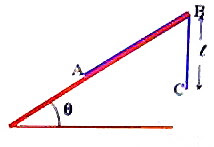(2) The adjoining figure shows a uniform chain of length L placed on a rough inclined plane of angle θ with a portion of length (of the chain) overhanging from the top edge B of the incline. The coefficient of friction between the chain and the inclined plane is μ. Now answer the following questions (a), (b) and (c):

(a) In the condition shown in the figure, the chain is just going to slide up the incline. Obtain an expression for the overhanging length of the chain in terms of the given parameters.

(b) If the chain were just going to slide down the incline, will the value of the overhanging length of the chain be different from the value obtained in part (a)?

Put a tick (√) mark against the correct option among (i), (ii) and (iii) given below:

(i) Yes_____ (ii) No_____ (iii) The answer will depend on the angle of the plane.

(c) If there is no friction between the chain and the inclined plane, determine the value of the overhanging length of the chain that will make it just start sliding down the plane.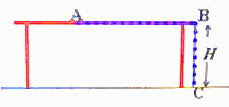The chain is now placed on a frictionless horizontal table of height H so that the portion AB of the chain is straight and is on the table (fig.) where as the remaining portion BC of length H is overhanging, with the end C just touching the ground. Now answer the following questions (d) and (e):

(d) When the chain is let free it slides along the table. Take the point A (initial position of the end of the chain) as the origin and write a differential equation for the acceleration of the chain at any instant during its motion.

(e) Solve the differential equation obtained in part (d) and determine the velocity of the chain when the end A just leaves the edge of the table.

(a) When the chain is just going to slide up the incline, the weight of the hanging portion of the chain tries to move the chain where as the component of the weight of the remaining portion (of length L) down the plane and the frictional force between the chain and the inclined plane try to oppose the motion. If the mass per unit length of the chain is m we have

ℓmg = (L )mg sinθ + μ(L )mg cosθ

[Note that the normal force exerted by the plane on the chain is (L )mg cosθ and μ is the coefficient of static friction].

Rearranging, (1+ sinθ + μ cosθ) = L(sinθ + μ cosθ)

Therefore, ℓ = L(sinθ + μ cosθ) /(1+ sinθ + μ cosθ)

(b) If the chain were just going to slide down the incline, the value of the overhanging length of the chain will be less than the value obtained in part (a). This is because of the fact that the frictional force will oppose the component of the weight of the portion of the chain that tries to move the chain down the plane.

(c) If there is no friction between the chain and the inclined plane, the value of the overhanging length of the chain that will make it just start sliding down the plane is obtained by equating the weight of the overhanging portion of the chain to the component of the weight of the remaining portion (of length L) down the plane:

ℓmg = (L )mg sinθ

This gives ℓ = (L sinθ) /(1+ sinθ)

(d) The force which drives the chain throughout its motion on the table top and is the weight (Hmg) of the vertical portion of the chain. Let the end of the chain move through a distance x from the origin (supposed to be at A) in time t. The acceleration (a) of the chain is given by

Hmg = (L x)m a

Or, Hmg = (L x)m (dv/dt) where v is the velocity of the portion of the chain on the table. The vertical portion also is moving and hence the total mass moved is that of length (L x).

This is the required differential equation for the acceleration (dv/dt) of the chain.

(e) The above equation can be rewritten as

Hg = (L x) (dv/dx) (dx/dt) = (L x) (dv/dx)v

Or, vdv = Hg dx/(L x)

Integrating, v2/2 = – Hg ln(L x) + C ----------(i)

where C is the constant of integration which can be found from the initial conditions.

Initially (at t = 0), when x = 0, v = 0. Substituting these in the above equation, we have

0 = – Hg ln(L) + C so that C = Hg ln(L)

Substituting this in Eq (i),

v2/2 = – Hg ln(L x) + Hg ln(L)

Therefore, v2 = 2 Hg ln[L/(L x)]

When the end of the chain just leaves the edge of the table, x = (L H) so that the velocity of the chain at the moment is given by

v = [2 Hg ln(L/H)]

## Friday, October 24, 2008

### Free Response Questions (for practice) on Newton’s Laws for AP Physics B & C

Today I give you two AP Physics practice questions on Newton’s laws of motion: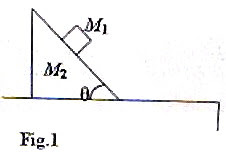(1) Free response question for AP Physics B:

Fig. 1 shows a small block of mass M1 placed on the rough inclined surface of a large wedge shaped block of mass M2 which is at rest on a rough horizontal table top. The coefficients of static friction and kinetic friction (between M1 and M2) are respectively μs and μk. Now answer the following questions (a), (b) and (c):

(a) Block M1 is just going to slide down. Draw the free body diagram for block M1 showing and identifying all the forces acting on it. (Represent M1 by a dot).

(b) What is the magnitude of the frictional force on M1 if it is at rest and does not show any sign of sliding down? Justify your answer.

(c) What is the magnitude of the frictional force on M1 if the mass M1 is sliding down? Justify your answer.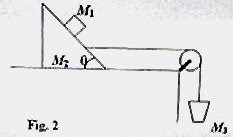Now suppose that there is no friction between the blocks M1 and M2 as well as between M2 and the table top. The system of masses M1 and M2 is connected to another mass M3 by a light inextensible string passing over a frictionless pulley of negligible mass as shown in fig.2. The mass M3 is such that the block M1 is prevented from sliding down along the block M2. Now answer the following questions (d) and (e):

(d) Determine the common acceleration of M1 and M2.

(e) Show that the condition for the block M1 not sliding along the block M2 is

M3 = (M1+M2)/(cot θ – 1)

The above question carries 15 points and you have about 17 minutes for answering it.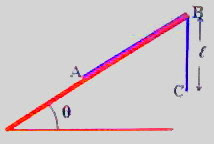(2) Free response question for AP Physics C:

The adjoining figure shows a uniform chain of length L placed on a rough inclined plane of angle θ with a portion of length (of the chain) overhanging from the top edge B of the incline. The coefficient of friction between the chain and the inclined plane is μ. Now answer the following questions (a), (b) and (c):

(a) In the condition shown in the figure, the chain is just going to slide up the incline. Obtain an expression for the overhanging length of the chain in terms of the given parameters.

(b) If the chain were just going to slide down the incline, will the value of the overhanging length of the chain be different from the value obtained in part (a)?Put a tick (√) mark against the correct option among (i), (ii) and (iii) given below:

(i) Yes_____ (ii) No_____ (iii) The answer will depend on the angle of the plane.

(c) If there is no friction between the chain and the inclined plane, determine the value of the overhanging length of the chain that will make it just start sliding down the plane.

The chain is now placed on a frictionless horizontal table of height H so that the portion AB of the chain is straight and is on the table (fig.) where as the remaining portion BC of length H is overhanging, with the end C just touching the ground. Now answer the following question (d) and (e):

(d) When the chain is let free it slides along the table. Take the point A (initial position of the end of the chain) as the origin and write a differential equation for the acceleration of the chain at any instant during its motion.

(e) Solve the differential equation obtained in part (d) and determine the velocity of the chain when the end A just leaves the edge of the table.

The above question carries 15 points and you are supposed to answer it in about 15 minutes.

AP Physics C aspirants should try to answer both questions. AP Physics B aspirants may omit part (e) of question No.2.

Try to answer these questions. I’ll be back soon with model answers for you.

## Sunday, October 19, 2008

### AP Physics B & C – Practice Questions (MCQ) on Conservation of Linear Momentum

In the post dted 24th September 2008 we had discussed the essential points to be remembered under Newton’s laws of motion. It was then stated that we will discuss the conservation of momentum separately. Today we will discuss some typical multiple choice questions involving the law of conservation of linear momentum.

As you know, Newton’s second law and third law lead to the law of conservation of momentum: The total momentum of an isolated system of interacting particles is conserved.

By isolated system you mean that the system is not acted on by external forces. There may be internal forces by which pairs of particles in the system interact thereby changing their individual momentum. But since the mutual forces for each pair are equal and opposite, the changes in momentum cancel in pairs and the total momentum remains unchanged. A typical case usually used to illustrate the law of conservation of momentum is the recoil of a gun when a bullet is fired. Initially, the bullet and the gun are at rest and hence the momentum of the system (of bullet and gun) is zero. On firing, the bullet moves forward because of the internal forces developed in the system. Since the final momentum of the system has to be zero, the forward momentum of the bullet has to be equal in magnitude to the backward (recoil) momentum of the gun at the instant of firing. If m and M represent the masses of the bullet and the gun and v and V the magnitudes of their velocities respectively, we have

mv + MV = 0

This gives the recoil velocity of the gun: V = mv/M

Now consider the following questions:

(1) A hose-pipe delivering water at the rate of 2.5 litre per second is held horizontally by a fire man. If the rate of flow of water is increased to 5 litre per second by increasing the speed of water, the fire man has to

(a) push forward with the same force as before

(b) push forward twice as hard

(c) push forward four times as hard

(d) push forward with half the force

(e) exert no horizontal force in both cases

Since the water jet has a forward momentum, the hose-pipe should have a backward momentum of the same magnitude (similar to the recoil momentum of a gun firing a bullet). The fire man has to exert a force in the direction of the water jet to hold the hose-pipe in position. When the rate of flow is doubled by increasing the speed of warer, the momentum imparted to the water jet per second is made four times sine the mass and velocity are doubled. Therefore, the fire man has to push forward four times as hard.

(2) A horizontal force F acts for a small time ∆t on an object of mass m1 initially at rest on a smooth horizontal surface (fig.). After the force has ceased, the object gets attached to another stationary object of mass m2 . What is the common velocity of the two objects moving together?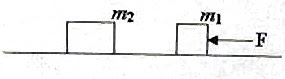(a) Zero

(b) F∆t m1/(m1+ m2)

(c) Fm1/(m1+ m2)

(d) F∆t m2/(m1+ m2)

(e) F∆t/(m1+ m2)

The impulse received by the object of mass m1 is F∆t. The momentum of this object therefore becomes F∆t. [Note that impulse is equal to the change in momentum. Since the mass m1 is initially at rest, its momentum becomes F∆t].

The initial momentum of the system (made of m1 and m2) is thus F∆t. The final momentum also is F∆t since the force has ceased. Therefore, we have

F∆t = (m1+ m2)v where ‘v’ is the common velocity of m1 and m2. This gives

v = F∆t/(m1+ m2)

(3) A shell fired from a cannon with velocity v at an angle of 45º with the horizontal explodes into two parts of equal mass at the highest point of its trajectory. One of the pieces retraces its path. What will be the speed of the other piece immediately after the explosion?

(a) 3v/2

(b) 3v/√2

(c) v/2

(d) v/√2

(e) 2v

At the highest point, the projectile (shell) has horizontal momentum only which is mvcosθ where m is the mass of the shell and θ is the angle of projection (45º here). Thus just before the explosion, the shell has momentum equal to mvcos45º = mv/√2

Since the total momentum of the two fragments immediately after the explosion must be equal to this, we have

mv/√2 = (m/2) vcos 45º + (m/2)v’ where v’ is the speed of the second piece.

Note that the first piece retraces its path and that’s why we have written its velocity as v cos 45º.

This gives

v/√2 = v/(2√2) + v’/2 from which v’ = 3v/√2

(4) An object initially at rest explodes into 3 fragments with momenta 30 kg ms–1, 40 kg ms–1 and 50 kg ms–1. What is the angle between the directions of flight of the fragments carrying momenta 30 kg ms–1 and 40 kg ms–1?

(a) Zero

(b) 30º

(d) 90º

(e) 120º

Since the total momentum of the fragments after the explosion has to be zero, the momentum 50 kg ms–1 of the 3rd fragment has to be equal and opposite to the resultant of the momenta of the other two fragments. In other words, the resultant of the momenta 30 kg ms–1and 40 kg ms–1 must be equal to 50 kg ms–1(represented by the vector OR in fig). Evidently, the angle between the momentum vectors OA and OB is 90º.

If you want you may write

502 = 302 + 402 + 2×30×40 cosθ

From this θ = 90º.

You will find a couple of solved questions in this section at physicsplus here as well as here

## Sunday, October 12, 2008

### AP Physics – Additional Questions (MCQ) on Newton’s Laws

As promised in the last post, I give below a few more multiple choice questions (with solution) on Newton’s Laws:

(1) The string of a simple pendulum inside a car (fig.) makes an angle of 30º with the vertical when the car accelerates along horizontal road AB. Pick out the correct option from the following:(a) The car is moving from A to B with acceleration approximately 5 ms–2

(b) The car is moving from B to A with acceleration approximately 5 ms–2

(c) The car is moving from B to A with acceleration approximately 10 ms–2

(d) The car is moving from A to B with acceleration approximately 7 ms–2

(e) The car is moving from B to A with acceleration approximately 7 ms–2The string of the pendulum makes an angle with the vertical because of the inertial force on the bob of the pendulum. Since the bob is deflected towards B, the car is moving from B to A. The inertial force on the bob is ma where m is the mass of the bob and a is the acceleration of the car. The bob is in equilibrium under the action of three forces: (i) weight mg of the bob, (ii) inertial force ma on the bob and (iii) tension T in the string. These can be represented by the triangle of forces ABC so that AB, BC and CA represent the forces mg, ma and T respectively. Therefore, we have

tan 30º = ma/mg from which a = 10/√2 ms–2, on substituting g = 10 ms–2 approximately. This works out to nearly 7 ms–2.

The correct option is (e).

(2) In the adjoining figure F1 represents the viscous drag force (due to air) on a car negotiating a circular curve on level ground. Which one among the other forces shown in the figure most appropriately represents the resultant horizontal frictional force acting on the tyres of the car?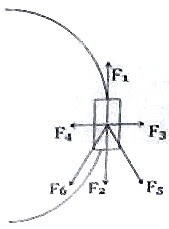(a) F1

(b) F2

(c) F3

(d) F4

(e) F5

The above question is meant for gauging your understanding of frictional force which moves a car along a road. Since the viscous drag force (due to air) on the car is represented by F1, the car is moving opposite to F1. At the poinf of contact on the road, the tyre is moving in the direction of F1 and the friction which moves the car forward is opposite to F1 and is to be represented by F2. The car is prevented from skidding by the component of friction acting radially inwards and is represented by F4. The resultant of F2 and F4 is represented by F6 which is the net horizontal frictional force acting on the tyres of the car.

(3) A boy slides a heavy box (fig.) along a horizontal rough surface by equal distances by applying a force in four different ways. Case (i): He pushes the box horizontally, applying the force along AB. Case (ii): He pulls the box horizontally, applying the force along CD. Case (iii): He pushes the box by applying the force along EF. Case (iv): He pulls the box by applying the force along GH. In which case/s he will have to do the least amount of work?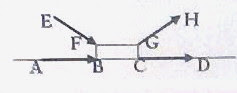(a) Case (ii)

(b) Case (iii)

(c) Case (iv)

(d) Cases (ii) and (iv)

(e) Cases (i), (ii) and (iv)

The boy need not do any work against gravity since he slides the box horizontally. Work has to be done against frictional force. Recall that the frictional force is μN where μ is the coefficient of friction and N is the normal force.Frictional force is least in case (iv) since the normal force is least and is equal to (mg F sinθ). In cases (i) and (ii) the normal force is mg. In case (iii) the normal force is (mg + F sinθ).

The boy will have to do the least amount of work in case (iv).

[Is it not easier to pull a lawn mower than to push it?]

(4) Two boys pull the ends of a light inextensible cord so that a tension of 50 N is developed in the cord. The forces exerted by them are

(a) 50 N and 50 N

(b) 100 N and 100 N

(c) 25 N and 25 N

(d) 30 N and 20 N

(e) given by options (c) and (d)

If one end of the cord is tied to a hook on the wall and one of the boys pulls the other end with a force of 50 N, what will be the tension in the cord? You will give the answer as 50 N with out any doubt. The second boy just functions as the hook and the answer to our problem is 50 N and 50 N [Option (a)].

(5) A block of wood is placed on a rough inclined plane whose inclination is gradually increased from zero. The frictional force between the block and the plane